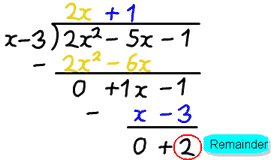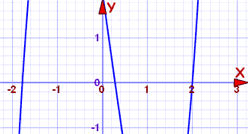# Remainder Theorem and Factor Theorem

Or: how to avoid Polynomial Long Division when finding factors

Do you remember doing division in Arithmetic?"7 divided by 2 equals 3 with a remainder of 1"

Each part of the division has names:Which can be rewritten as a sum like this:## Polynomials

Well, we can also divide polynomials.

f(x) ÷ d(x) = q(x) with a remainder of r(x)

But it is better to write it as a sum like this:Like in this example using Polynomial Long Division:

### Example: 2x2−5x−1 divided by x−3

• f(x) is 2x2−5x−1
• d(x) is x−3After dividing we get the answer 2x+1, but there is a remainder of 2.

• q(x) is 2x+1
• r(x) is 2

In the style f(x) = d(x)·q(x) + r(x) we can write:

2x2−5x−1 = (x−3)(2x+1) + 2

But you need to know one more thing:

The degree of r(x) is always less than d(x)

Say we divide by a polynomial of degree 1 (such as "x−3") the remainder will have degree 0 (in other words a constant, like "4").

We will use that idea in the "Remainder Theorem":

## The Remainder Theorem

When we divide f(x) by the simple polynomial x−c we get:

f(x) = (x−c)·q(x) + r(x)

x−c is degree 1, so r(x) must have degree 0, so it is just some constant r :

f(x) = (x−c)·q(x) + r

Now see what happens when we have x equal to c:

f(c) =(c−c)·q(c) + r
f(c) =(0)·q(c) + r
f(c) =r

So we get this:

The Remainder Theorem:

When we divide a polynomial f(x) by x−c the remainder is f(c)

So to find the remainder after dividing by x-c we don't need to do any division:

Just calculate f(c).

Let us see that in practice:

### Example: The remainder after 2x2−5x−1 is divided by x−3

(Our example from above)

We don't need to divide by (x−3) ... just calculate f(3):

2(3)2−5(3)−1 = 2x9−5x3−1
= 18−15−1
= 2

And that is the remainder we got from our calculations above.

We didn't need to do Long Division at all!

### Example: The remainder after 2x2−5x−1 is divided by x−5

Same example as above but this time we divide by "x−5"

"c" is 5, so let us check f(5):

2(5)2−5(5)−1 = 2x25−5x5−1
= 50−25−1
= 24

The remainder is 24

Once again ... We didn't need to do Long Division to find that.

## The Factor Theorem

Now ...

What if we calculate f(c) and it is 0?

... that means the remainder is 0, and ...

... (x−c) must be a factor of the polynomial!

We see this when dividing whole numbers. For example 60 ÷ 20 = 3 with no remainder. So 20 must be a factor of 60.

### Example: x2−3x−4

f(4) = (4)2−3(4)−4 = 16−12−4 = 0

so (x−4) must be a factor of x2−3x−4

And so we have:

The Factor Theorem:

When f(c)=0 then x−c is a factor of f(x)

And the other way around, too:

When x−c is a factor of f(x) then f(c)=0

## Why Is This Useful?

Knowing that x−c is a factor is the same as knowing that c is a root (and vice versa).

The factor "x−c" and the root "c" are the same thing

Know one and we know the other

For one thing, it means that we can quickly check if (x−c) is a factor of the polynomial.

### Example: Find the factors of 2x3−x2−7x+2

The polynomial is degree 3, and could be difficult to solve. So let us plot it first:The curve crosses the x-axis at three points, and one of them might be at 2. We can check easily:

f(2) = 2(2)3−(2)2−7(2)+2
= 16−4−14+2
= 0

Yes! f(2)=0, so we have found a root and a factor.

So (x−2) must be a factor of 2x3−x2−7x+2

How about where it crosses near −1.8?

f(−1.8) = 2(−1.8)3−(−1.8)2−7(−1.8)+2
= −11.664−3.24+12.6+2
= −0.304

No, (x+1.8) is not a factor. We could try some other values near by and maybe get lucky.

But at least we know (x−2) is a factor, so let's use Polynomial Long Division:

2x2+3x−1
x−2)2x3− x2−7x+2
2x3−4x2
3x2−7x
3x2−6x
−x+2
−x+2
0

As expected the remainder is zero.

Better still, we are left with the quadratic equation 2x2+3x−1 which is easy to solve.

It's roots are −1.78... and 0.28..., so the final result is:

2x3−x2−7x+2 = (x−2)(x+1.78...)(x−0.28...)

We were able to solve a difficult polynomial.

## Summary

The Remainder Theorem:

• When we divide a polynomial f(x) by x−c the remainder is f(c)

The Factor Theorem:

• When f(c)=0 then x−c is a factor of f(x)
• When x−c is a factor of f(x) then f(c)=0

Challenging Questions: 1 2 3 4 5 6